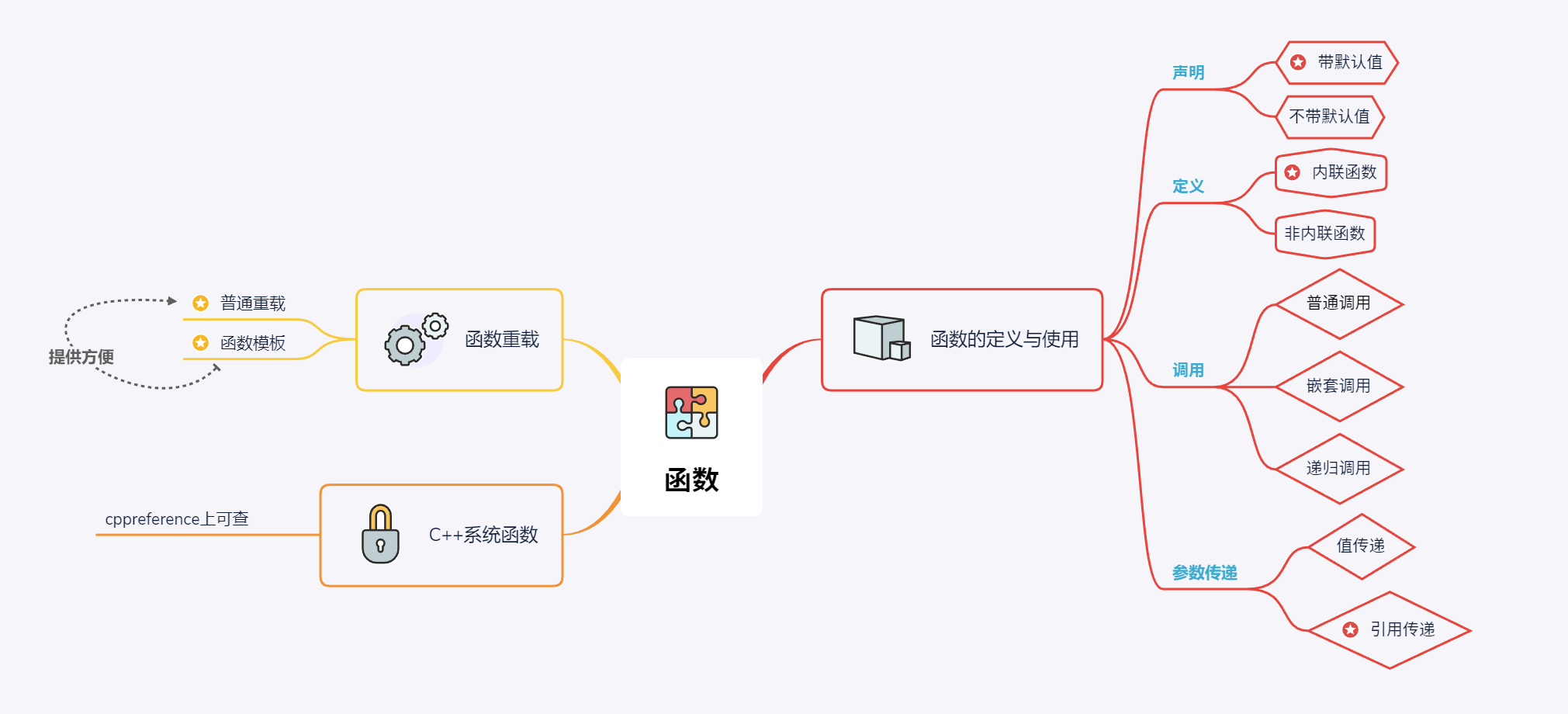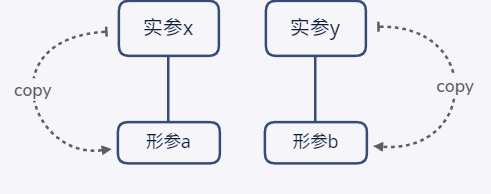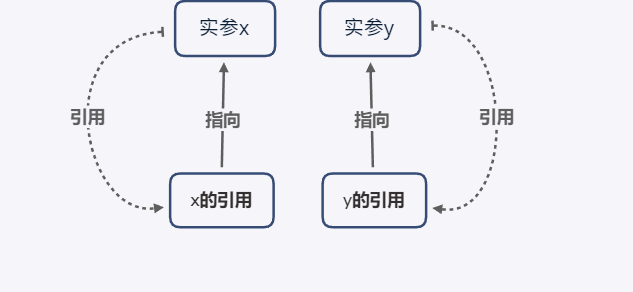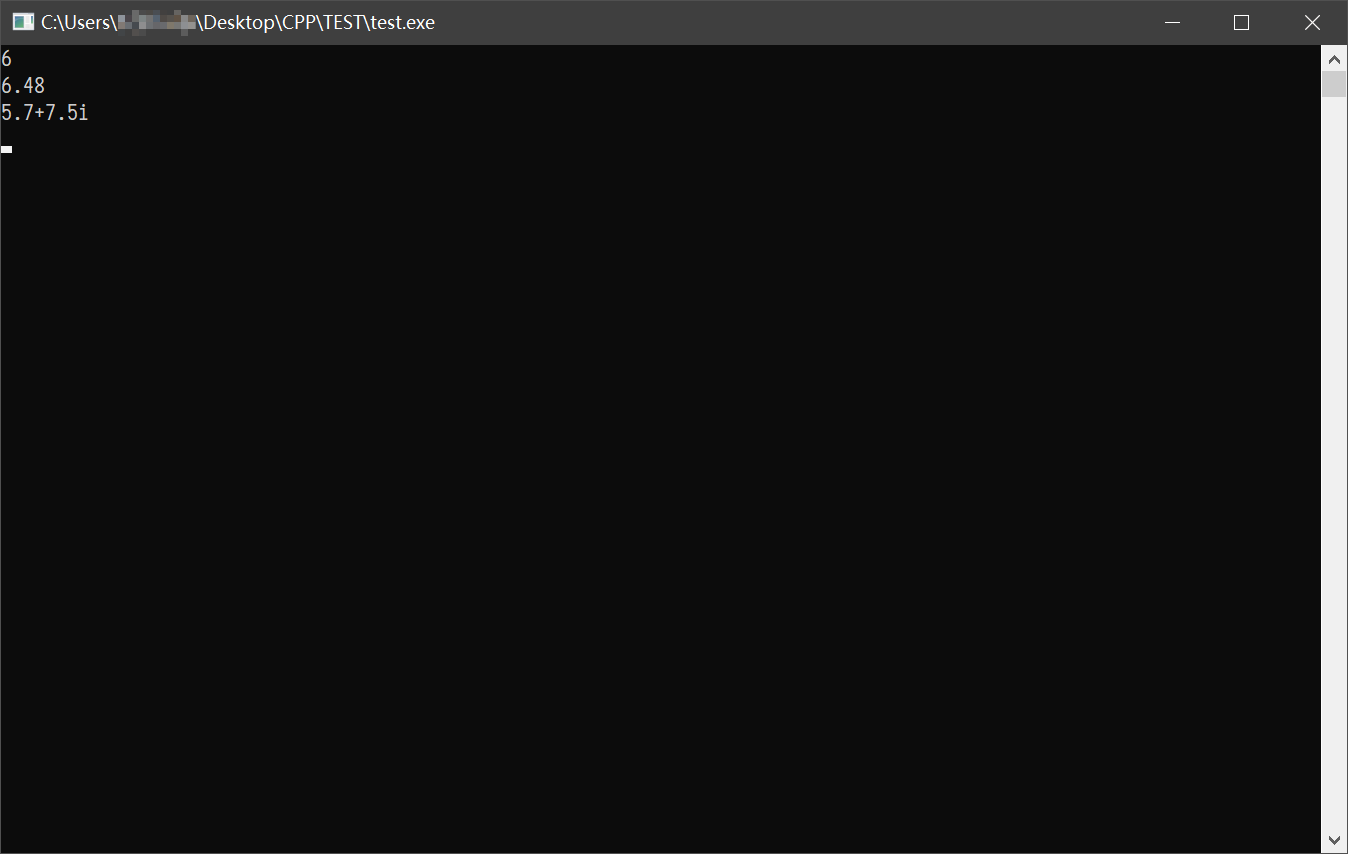# C++第三章复习与总结（思维导图分享）## 函数的定义与使用

### 带默认值的函数

#include <iostream>

using namespace std;

int add(int a = 5, int b = 6)
{
return a + b;
}

int main(void)
{
return 0;
}

#include <iostream>

using namespace std;

int add(int a = 5, int b = 6);

int main(void)
{
return 0;
}

int add(int a, int b)  //add(int a = 5,b = 6)为错误写法
{
return a + b;
}

### 内联函数

C++中添加了一个新的关键字inline，在函数定义前加上即可将函数嵌入所有调用函数的地方，以此来消除调用函数时产生的时间开销，适用于简单且规模小的函数。

### 参数传递

#### 值传递

C++中的值传递与C语言中的值传递并无两样。在发生普通的函数调用时，系统会给形参分配空间并用实参的值给形参初始化。#### 引用传递## 函数重载

C++中的函数重载允许我们使用相同的函数名却使用不同数据类型的数据与返回值，在进行函数调用的时候，编译器会自动根据数据类型选择合适的函数。

### 普通的函数重载

#include <complex>
#include <iostream>
using namespace std;

int main()
{
int a = 1, b = 5;
double x = 1.12, y = 5.36;
complex<double> m{2.2, 5.4};
complex<double> n{3.5, 2.1};
cout << add(a, b) << endl;
cout << add(x, y) << endl;
cout << add(m, n).real() << "+" << add(m, n).imag() << "i" << endl;
return 0;
}

int add(int a, int b)
{
return a + b;
}

double add(double a, double b)
{
return a + b;
}

complex<double> add(complex<double> a, complex<double> b)
{
return a + b;
}### 用函数模板实现函数重载

C++中有一个更方便的方法，就是使用函数模板。但是函数模板有它的缺点，就是只有除了数据类型不同，其他代码一模一样的函数才能使用函数模板合并，我们上文中例子里的三个函数刚好都符合，那么就可以使用函数模板将他们合并。

#include <complex>
#include <iostream>
using namespace std;

template <typename T>
T add(T a, T b)
{
return a + b;
}

int main()
{
int a = 1, b = 5;
double x = 1.12, y = 5.36;
complex<double> m{2.2, 5.4};
complex<double> n{3.5, 2.1};
cout << add(a, b) << endl;
cout << add(x, y) << endl;
cout << add(m, n).real() << "+" << add(m, n).imag() << "i" << endl;
cin.get();
return 0;
}

## C++系统函数

posted @ 2019-03-17 23:53 KOKODA 阅读(...) 评论(...) 编辑 收藏